# ¶ FluidNC Spindle Speed Mapping

FluidNC includes a flexible way to control the relationship between GCode S values and spindle speeds. Previously the mapping from S values to spindle speed was done with a few global settings named GCode/MinS, GCode/MaxS, Spindle/PWM/Frequency, Spindle/PWM/Off, Spindle/PWM/Min, and Spindle/PWM/Max. The old scheme had a minumum speed for nonzero S values less than Spindle/PWM/Min, followed by a linear ramp from MinS to MaxS. The code had stubs for a "piecewise linear" multi-segment mapping curve, but it was not implemented.

In the new scheme, each spindle (several can be configured at once) has its own multi-segment "speed map" that can do everything that the old scheme could do, and more, including piecewise-linear mapping. The basis of the scheme is a text string that lists "inflection points" in a piecewise linear curve, as in this example:

speed_map: 0=0% 0=20% 4000=20% 8000=30% 16000=100%

Each entry is of the form Svalue=spindle% . Svalue is the value of GCode "S word" as in "M3 S1000". spindle% is a percentage from 0 to 100% of the spindle's maximum speed or power. It is tempting to think of both Svalues and spindle speed as RPM, but that is often incorrect. For example, a Laser "spindle" does not revolve, so "Revolutions Per Minute" is wrong. At the hardware level where spindles are actually controlled, the control signal can take many different forms with different units. For example, a voltage-controlled spindle might set the speed from a 0 to 10V signal, and the hardware that generates that 0-10V signal might in turn be controlled by a number from 0 to 1023. The right way of think of spindle% is that 100% corresponds to the maximum value of the control signal, whatever it might be.

Each entry is a point in a graph that converts GCode S numbers to spindle speed or power. If S=0, the spindle speed is set to the first percentage in the list - typically 0% but that is not required. If S is not 0, then the code looks for the first point whose Svalue is greater than S and uses the segment just to the left of that point. The spindle speed is interpolated within that segment.

Speeds requested that are a value higher than the highest speed specified in the speed map with use the highest percentage. So if your highest value is 18000=75% and you request a speed of 20000, it will still be 75%.

## ¶ Piecewise Linear Curve with Minimum Speed Shelf

speed_map: 0=0% 0=20% 4000=20% 8000=30% 16000=100%

corresponds to this graph of S values (horizontal axis) to spindle% (vertical axis)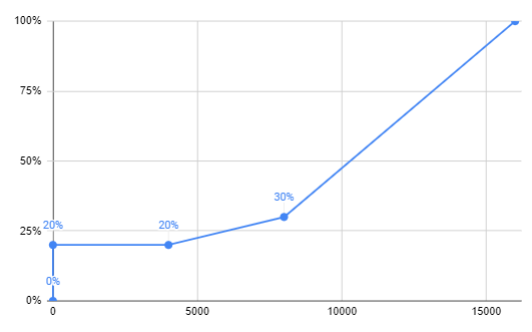In this example, S=0 uses 0%, S>0 and <4000 use 20%, S>=4000 and <8000 is a linear ramp from 20% to 30%, S>=8000 and < 16000 is a linear ramp from 30% to 100%. If S>= 16000 uses 100%, which is the percentage in the final entry. The "shelf" from (just above) S0 to S4000 is a common "minimum speed" feature that is often used for spindles that lose torque, or cannot run at all, or tend to overheat at low speeds. Switching directly from off to some minimum speed can prevent damage to bits and motors.

## ¶ Linear Curve with Dead Zone

speed_map: 0=0% 1000=0% 10000=100%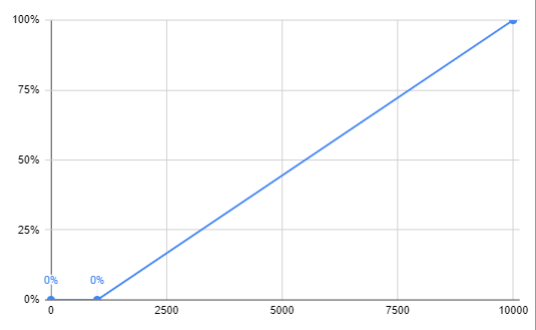In this example, the spindle speed or power is 0% until S=1000, then ramps up linearly to full speed when S=10000, remaining at full speed if S>10000.

## ¶ Relay or Off/On Spindle

You can specify that any non-zero S value turns on the spindle with

speed_map: 0=0% 0=100% 1=100%

The transition from off (0%) to full-on (100%) happens just after 0. The graph of this is trivial and not shown; it is just a vertical line from 0 to 100% at S=0.

## ¶ Fully Linear Spindle

speed_map: 0=0% 10000=100%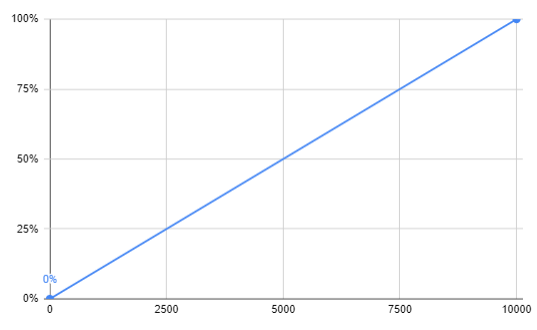This is a simple case where the spindle speed/power ramps up linearly between S=0 and S=10000, with no special behavior for low speeds.

## ¶ Nonzero Spindle% at S=0

The spindle% for the first entry need not be 0%. You might have a spindle where S=0 requires a nonzero value for some control signal.

speed_map: 0=20% 10000=100%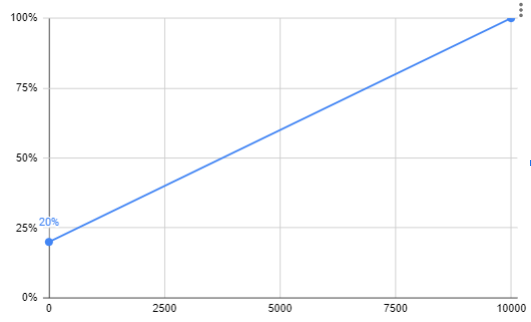A curve like this might be used with a 4-20mA current loop, for example.

## ¶ Maximum Spindle% < 100%

Suppose that you have a spindle whose maximum speed is 24000 RPM when its control signal is 100%, but you wish to limit the speed to, say, 18000 RPM because, say, the bearings overheat at higher speeds.

speed_map: 0=0% 0=25% 6000=25% 18000=75%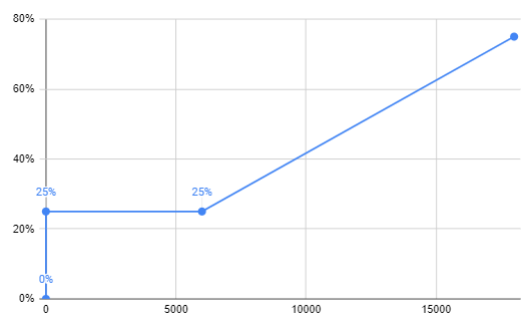Notice that the spindle speed/power stops at 75%. Any S values greater than 180000 will limit to 75%.